## Tag: complex

#### Beware of square roots and exponentials!

In the last post I asked you the question, what is wrong with the following proof.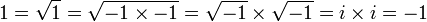As some of you noticed, the answer lies in the fact that the identity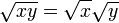is only true for positive real numbers x and y. In general one has to be careful with the identity

(xy)a = xaya

for non-integer a and non-positive x and y.

I posted this question because, although it ought to be, it is not always taught to students when complex numbers are introduced. Once students are taught complex numbers they feel that, now that they are free to take the square root of any real number, they don’t have to pay attention anymore to what they are doing. They apply the rules blindly, resting in false comfort that everything is now fine.

Another pitfall with complex numbers, that even educated people are not always aware of, is the fact that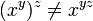if y and z are complex numbers, even for positive, real x. If it were we, could prove the following (here I assume that you are familiar with the complex exponential function).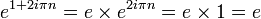This makes

(e1 + 2iπn)2iπn = (e)2iπn = 1

But, if the above identity were true, the last line would be equal to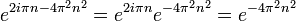Which, in general, is a positive real number not equal to 1.

#### Another proof that -1=1

Here is a little maths teaser for you. Since I was a student, I always loved those “proofs” that zero equals one. Of course, most of the time, this was achieved by sneakily dividing by zero somewhere along the way.

But yesterday I came across a proof that used a different, slightly more subtle trick and uses complex numbers. I apologise to any reader not familiar with complex numbers. Anyone interested can find a quick introduction here.

Enough introduction, here is the “proof”:Looks OK, but it can’t be right of course. So where is the error in this equation? Can you find out?

Update:
You can find the answer here.